## Molecular biomarker candidates of acute kidney injury in zero-hour renal transplant needle biopsies.

Reka Korbély(1,3), Julia Wilflingseder(1,2), Paul Perco(2,4), Alexander Kainz(1,2), Robert M. Langer(3), Bernd Mayer(4), Rainer Oberbauer(1,2)

1- Department of Nephrology, KH Elisabethinen, Linz, Austria

2- Department of Nephrology, Medical University of Vienna, Austria

3- Department of Transplantation and Surgery, Semmelweis University, Budapest, Hungary

4- emergentec biodevelopment GmbH, Vienna, Austria

// Tables
// Figures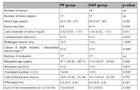Table 1:

Demographic data of transplant donors and recipients stratified by treatment assignment. Continuous data are provided as median (1st quartile, 3rd quartile), categorical data are given as counts.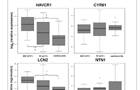Figure 1:

Box-Whisker-Plots of the four biomarkers in the tubulointerstitium. Boxplots show the median and the 1.5 interquartile range of the log2 (relative expression) measured in the qRT-PCR experiment. A value of zero equates same expression level like in the reference RNA.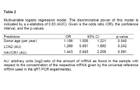Table 2:

Multivariable logistic regression model. The discriminative power of this model is indicated by a c-statistics of 0.83 (AUC). Given is the odds ratio (OR), the confidence interval, and the p-values.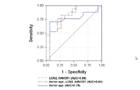Figure 2:

ROC curves: Discrimination for DGF after transplantation using donor age (solid blue line), expression features (dashed red line), or the combination of both (dashed green line).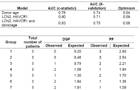Table 3:

Discrimination of the models and optimism (3A) derived from the re-sampling procedure (34-fold cross validation). Calibration of the DGF prediction model by the Hosmer-Lemeshow goodness of fit test (3B). The expected to observed number of cases in each of the deciles of patients were not statistically different suggesting good calibration (p=0.76, chi-square test).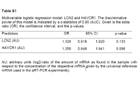Table S1:

Multivariable logistic regression model: LCN2 and HAVCR1. The discriminative power of this model is indicated by a c-statistics of 0.80 (AUC). Given is the odds ratio (OR), the confidence interval, and the p-values.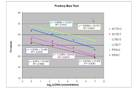Figure S1:

Bias Test of pre-amplification technique: Dilution series from a microdissected nephrectomy sample (T: Tubulointerstium, G: glomeruli part) were measured with qRT-PCR for ACTB (ß-actin), LCN2 (lipocalin 2) and PPIA (Cyclophilin A) after a 10 cycle pre-amplification. All regression lines show excellent correlation between a wide dynamic range (125ng/µl to 1ng/µl cDNA concentration before pre-amplification). The regression lines of PPIA and LCN2 show similar slopes, therefore we decided to use PPIA as endogenous control gene.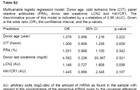Table S2:

Multivariable logistic regression model: Donor age, cold ischemic time (CIT), panel reactive antibodies (PRA), donor last creatinine, LCN2 and HAVCR1. The discriminative power of this model is indicated by a c-statistics of 0.86 (AUC). Given is the odds ratio (OR), the confidence interval, and the p-values.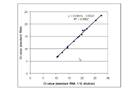Figure S2:

Bias test for reverse transcription, pre-amplification and realtime PCR of the reference RNA: Correlation of fourteen different genes in the standard RNA (Stratagene Human reference RNA) is almost one, suggesting reference RNA is an excellent calibrator.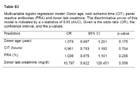Table S3:

Multivariable logistic regression model: Donor age, cold ischemic time (CIT), panel reactive antibodies (PRA) and donor last creatinine. The discriminative power of this model is indicated by a c-statistics of 0.85 (AUC). Given is the odds ratio (OR), the confidence interval, and the p-values.

SCIENTIFIC COLLABORATIONS